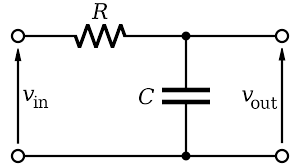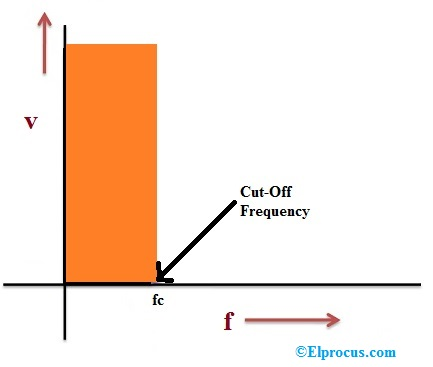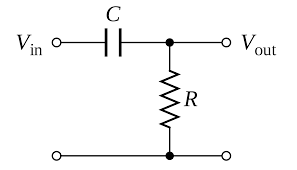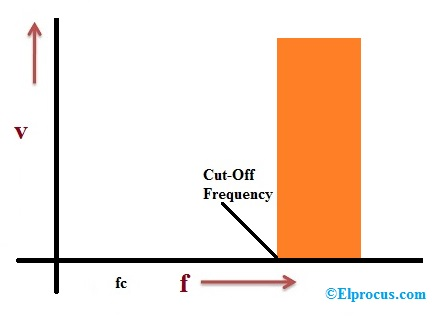# Differences between Low Pass Filter and High Pass Filter

The main disparity between LPF-low pass filter and high pass filter-HPF is the frequency range which they exceed. An HPF (high pass filter) is one kind of circuit which permits the high frequency and blocks low frequency for flowing through it. In the same way, an LPF (low pass filter) is one kind of circuit which permits the low-frequency and blocks high-frequency for flowing through it. In filters, the cut off frequency will decide the range of high frequency as well as low frequency. Before discussing the filter operational method, we have to know the required components of these filters. The LPF and HPF can be designed with electronic components like resistor, amplifier, capacitor, etc.

## What is Low Pass Filter and High Pass Filter?

An overview of low pass filter and high pass filter with differences are discussed below.

### Low Pass Filter

The circuit diagram of the low pass filter is shown below. The circuit of LPF can be built with a resistor as well as a capacitor in series so that the output can be achieved. Once the input is given to the circuit of the LPF, then the resistance will give a stable obstacle, however, the capacitor position will have an effect on the output signal.Low Pass Filter

If the high-frequency signal is applied to the LP circuit, thus it will exceed from resistance which will offer the standard resistance, however, the resistance accessible from the capacitor will be nothing. This is due to the resistance offered from the capacitor toward the high-frequency signal will be zero whereas the low-frequency signal is unlimited.

From the above circuit of the low pass filter, It is understandable that once the high-frequency signal comes LPF circuit afterward the capacitor will permit it to flow as well as it will pass to the GND. In this state, the o/p voltage achieved will be zero because the whole voltage is supplied to ground.
However when the low-frequency signal goes through the LPF circuit then the output will be generated, as the resistance will give a similar obstacle like the high-frequency signal although the capacitor will offer infinite resistance.Low Pass Filter Response

Therefore, in this state, the signal cannot flow through the lane of the capacitor. So the total low-frequency signal will be supplied to the output terminal.

### High Pass Filter

The circuit diagram of the high pass filter is shown below. An HPF blocks the low-frequency signals & allows just high-frequency signals for flowing through it. Even though it provides reduction to high-frequency signal also however the attenuation issue is so little that it can be ignored. This can be obtainable by the resistor and capacitor characteristics.High Pass Filter

When the input signal is applied to the capacitor, then the voltage across the resistor can be achieved because of the o/p voltage. The combination of the resistance of the resistor as well as a capacitor can be called reactance.

Xc = 1/2пfc

From the above equation, we can conclude that the reactance will be inversely proportional to the cut-off frequency. When the input signal’s frequency is superior, then the reactance will be low. Similarly, when the input signal’s frequency is low, then the reactance will be low.High Pass Filter Response

## Difference between Low Pass Filter and High Pass Filter

The difference between low pass filter and high pass filter mainly includes definition, circuit architecture, significance, operating frequency, and applications.

 Low Pass Filter High Pass Filter LPF circuit allows the frequency underneath cut-off frequency for flowing through it. HPF circuit allows the frequencies over cut-off frequency for flowing through it. It can be built with a resistor which is followed by a capacitor. It can be built with a capacitor which is followed by a resistor. It is important in eliminating the aliasing effect. It is important whenever the distortion occurs because of low-frequency signal like noise is to be detached. It is lesser than the cut-off frequency. It is higher than the cut-off frequency. The LPF can be used as an anti-aliasing filter in communication circuits. The HPF can be used in amplifiers like low noise, audio, etc.

Thus, this is all about the main differences between the low pass filter and high pass filter, circuit working, and low pass and high pass filter graphs. From the above information, finally, we can conclude that the HPF circuit allows the high-frequency signals which are high than the cut-off frequency whereas the LPF circuit allows the low-frequency signals which are low then the cut-off frequency. In the above low pass and high pass filter experiment, the two filters we have discussed in the above are passive filters because the circuits of these filters utilize passive components. The signal gain can be enhanced with the help of amplifiers in the circuit so that it will become an active filter. Here is a question for you, what are the applications of LPF and HPF?